# Calculating Forces Required to Cause Prescribed Motion of a Particle (Part - 1) Civil Engineering (CE) Notes | EduRev

## Civil Engineering (CE) : Calculating Forces Required to Cause Prescribed Motion of a Particle (Part - 1) Civil Engineering (CE) Notes | EduRev

The document Calculating Forces Required to Cause Prescribed Motion of a Particle (Part - 1) Civil Engineering (CE) Notes | EduRev is a part of the Civil Engineering (CE) Course Introduction to Dynamics and Vibrations- Notes, Videos, MCQs.
All you need of Civil Engineering (CE) at this link: Civil Engineering (CE)

3.2 Calculating forces required to cause prescribed motion of a particle

Newton’s laws of motion can be used to calculate the forces required to make a particle move in a particular way.

We use the following general procedure to solve problems like this

(1) Decide how to idealize the system (what are the particles?)
(2) Draw a free body diagram showing the forces acting on each particle
(3) Consider the kinematics of the problem. The goal is to calculate the acceleration of each particle in the system – you may be able to start by writing down the position vector and differentiating it, or you may be able to relate the accelerations of two particles (eg if two particles move together, their accelerations must be equal).
(4) Write down F=ma for each particle.
(5) If you are solving a problem involving a massless frames (see, e.g. Example 3, involving a bicycle with negligible mass) you also need to write down MC = 0 about the particle.

(5) Solve the resulting equations for any unknown components of force or acceleration (this is just like a statics problem, except the right hand side is not zero).

It is best to show how this is done by means of examples.

Example 1: Estimate the minimum thrust that must be produced by the engines of an aircraft in order to take off from the deck of an aircraft carrier

We will estimate the acceleration required to reach takeoff speed, assuming the aircraft accelerates from zero speed to takeoff speed along the deck of the carrier, and then use Newton’s laws to deduce the force.

Data/ Assumptions:

1. The flight deck of a Nimitz class aircraft carrier is about 300m long but only a fraction of this is used for takeoff (the angled runway is used for landing). We will take the length of the runway to be d=200m
2. We will assume that the acceleration during takeoff roll is constant.
3. We will assume that the aircraft carrier is not moving (this is wrong – actually the aircraft carrier always moves at high speed during takeoff. We neglect motion to make the calculation simpler)
4. The FA18 Super Hornet is a typical aircraft used on a carrier – it has max catapult weight of m=15000kg
5. The manufacturers are somewhat reticent about performance specifications for the Hornet but vt = 150 knots (77 m/s) is a reasonable guess for a minimum controllable airspeed for this aircraft.

Calculations:

1. Idealization: We will idealize the aircraft as a particle. We can do this because the aircraft is not rotating during takeoff.

2. FBD: The figure shows a free body diagram. FT represents the (unknown) force exerted on the aircraft due to its engines

3. Kinematics: We must calculate the acceleration required to reach takeoff speed. We are given (i) the distance to takeoff d, (ii) the takeoff speed vt and (iii) the aircraft is at rest at the start of the takeoff roll. We can therefore write down the position vector r and velocity v of the aircraft at takeoff, and use the straight line motion formulas for r and v to calculate the time t to reach takeoff speed and the acceleration a. Taking the origin at the initial position of the aircraft, we have that, at the instant of takeoff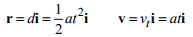This gives two scalar equations which can be solved for a and t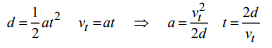4. EOM: The vector equation of motion for this problem is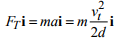5. Solution: The i component of the equation of motion gives an equation for the unknown force in terms of known quantities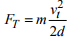Substituting numbers gives the magnitude of the force as F=222 kN. This is very close, but slightly greater than, the 200kN (44000lb) thrust quoted on the spec sheet for the Hornet. Using a catapult to accelerate the aircraft, speeding up the aircraft carrier, and increasing thrust using an afterburner buys a margin of safety.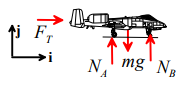Example 2: Mechanics of Magic! You have no doubt seen the simple `tablecloth trick’ in which a tablecloth is whipped out from underneath a fully set table (if not, you can watch it at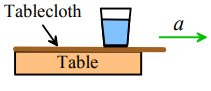In this problem we shall estimate the critical acceleration that must be imposed on the tablecloth to pull it from underneath the objects placed upon it.

We wish to determine conditions for the tablecloth to slip out from under the glass. We can do this by calculating the reaction forces acting between the glass and the tablecloth, and see whether or not slip will occur. It is best to calculate the forces required to make the glass move with the tablecloth (i.e. to prevent slip), and see if these forces are big enough to cause slip.

1. Idealization: We will assume that the glass behaves like a particle (again, we can do this because the glass does not rotate)

2. FBD. The figure shows a free body diagram for the glass. The forces include (i) the weight; and (ii) the normal and tangential components of reaction at the contact between the tablecloth and the glass. The normal and tangential forces must act somewhere inside the contact area, but their position is unknown. For a more detailed discussion of contact forces see Sects 2.4 and 2.5.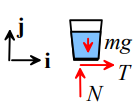3. Kinematics We are assuming that the glass has the same acceleration as the tablecloth. The table cloth is moving in the i direction, and has magnitude a. The acceleration vector is therefore a = ai .

4. EOM. Newton’s laws of motion yield

F = ma ⇒ Ti + (N - mg)j = mai

5. Solution: The i and j components of the vector equation must each be satisfied (just as when you solve a statics problem), so that

T = ma    N - mg = 0 ⇒N mg

Finally, we must use the friction law to decide whether or not the tablecloth will slip from under the glass. Recall that, for no slip, the friction force must satisfy

|T| < μN

where μ is the friction coefficient. Substituting for T and N from (5) shows that for no slip

|a| < μg

To do the trick, therefore, the acceleration must exceed μg. For a friction coefficient of order 0.1, this gives an acceleration of order 1m / s2 . There is a special trick to pulling the tablecloth with a large acceleration – but that’s a secret.

Example 3: Bicycle Safety. If a bike rider brakes too hard on the front wheel, his or her bike will tip over In this example we investigate the conditions that will lead the bike to capsize, and identify design variables that can influence these conditions.

If the bike tips over, the rear wheel leaves the ground. If this happens, the reaction force acting on the wheel must be zero – so we can detect the point where the bike is just on the verge of tipping over by calculating the reaction forces, and finding the conditions where the reaction force on the rear wheel is zero.

1. Idealization: a. We will idealize the rider as a particle (apologies to bike racers – but that’s how we think of you…). The particle is located at the center of mass of the rider. The figure shows the most important design parameters- these are the height of the rider’s COM, the wheelbase L and the distance of the COM from the rear wheel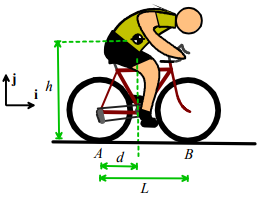b. We assume that the bike is a massless frame. The wheels are also assumed to have no mass. This means that the forces acting on the wheels must satisfy F = 0 and M = 0 - and can be analyzed using methods of statics. If you’ve forgotten how to think about statics of wheels, you should re-read the notes on this topic – in particular, make sure you understand the nature of the forces acting on a freely rotating wheel (Section 2.4.6 of the reference notes).
c. We assume that the rider brakes so hard that the front wheel is prevented from rotating. It must therefore skid over the ground. Friction will resist this sliding. We denote the friction coefficient at the contact point B by μ .
d. The rear wheel is assumed to rotate freely.
e. We neglect air resistance.

2. FBD. The figure shows a free body diagram for the rider and for the bike together. Note that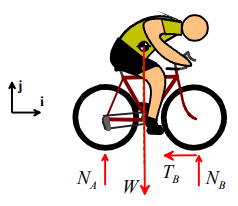a. A normal and tangential force acts at the contact point on the front wheel (in general, both normal and tangential forces always act at contact points, unless the contact happens to be frictionless). Because the contact is slipping it is essential to draw the friction force in the correct direction – the force must resist the motion of the bike;

b. Only a normal force acts at the contact point on the rear wheel because it is freely rotating, and behaves like a 2-force member.

3. Kinematics The bike is moving in the i direction. As a vector, its acceleration is therefore a = ai , where a is unknown.

4. EOM: Because this problem includes a massless frame, we must use two equations of motion (F = ma and MC = 0). It is essential to take moments about the particle (i.e. the rider’s COM).

F = ma gives TBi+(NA + NB -W)j =  mai

It’s very simple to do the moment calculation by hand, but for those of you who find such calculations unbearable here’s a Mupad script to do it. The script simply writes out the position vectors of points A and B relative to the center of mass as 3D vectors, writes down the reactions at A and B as 3D vectors, and calculates the resultant moment (we don’t bother including the weight, because it acts at the origin and so exerts zero moment)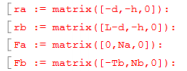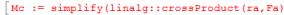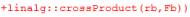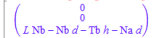The two nonzero components of F = ma and the one nonzero component of MC = 0 give us three scalar equations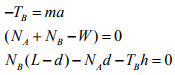We have four unknowns – the reaction components NA,NB,TB and the acceleration a so we need another equation. The missing equation is the friction law

TB = μNB

5. Solution: Here’s the solution with Mupad. It’s easy to get the same answer by hand as well.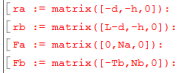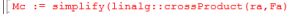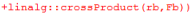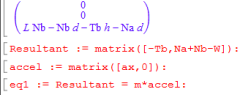We are interested in finding what makes the reaction force at A go to zero (that’s when the bike is about to tip). So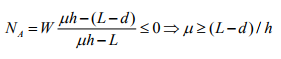This tells us that the bike will tip if the friction coefficient exceeds a critical magnitude, which depends on the geometry of the bike. The simplest way to design a tip-resistant bike is to make the height of the center of mass h small, and the distance (L-d) between the front wheel and the COM as large as possible.

Example 4: A stupid problem that you might find in the FE professional engineering exam. The purpose of this problem is to show what you need to do to solve problems involving more than one particle.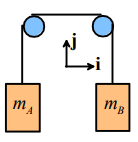Two weights of mass mA and mB are connected by a cable passing over two freely rotating pulleys as shown. They are released, and the system begins to move. Find an expression for the tension in the cable connecting the two weights.

1. Idealization – The masses will be idealized as particles; the cable is inextensible and the mass of the pulleys is neglected. This means the internal forces in the cable, and the forces acting between cables/pulleys must satisfy F = 0 and M = 0, and we can treat them as though they were in static equilibrium.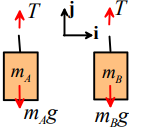2. FBD – we have to draw a separate FBD for each particle. Since the pulleys and cable are massless, the tension T in the cable is constant.

3. Kinematics We know that both masses must move in the j direction. We also know that the masses always move at the same speed but in opposite directions. Therefore, their accelerations must be equal and opposite. We can express this mathematically as

aAj = aB

4. EOM: We must write down two equations of motion, as there are two masses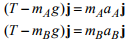We now have three equations for three unknowns (the unknowns are , aA aB and T).

5. Solution: As paid up members of ALE (the Academy of Lazy Engineers) we use Mupad to solve the equations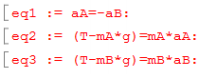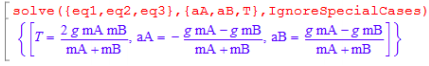So the tension in the cable is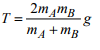We pass!

Example 5: Another stupid FE exam problem: The figure shows a small block on a rotating bar. The contact between the block and the bar has friction coefficient μ . The bar rotates at constant angular speed ω . Find the critical angular velocity that will just make the block start to slip when θ = 0 . Which way does the block slide?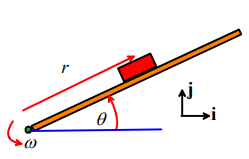The general approach to this problem is the same as for the Magic trick example – we will calculate the reaction force exerted by the bar on the block, and see when the forces are large enough to cause slip at the contact. We analyze the motion assuming the slip does not occur, and then find out the conditions where this can no longer be the case.

1. Idealization – We will idealize the block as a particle. This is dangerous, because the block is clearly rotating. We hope that because it rotates at constant rate, the rotation will not have a significant effect – but we can only check this once we know how to deal with rotational motion.

2. FBD: The figure shows a free body diagram for the block. The block is subjected to a vertical gravitational force, and reaction forces at the contact with the bar. Since we have assumed that the contact is not slipping, we can choose the direction of the tangential component of the reaction force arbitrarily. The resultant force on the block is

F = T cosθ - N sinθ) i + (N cosθ + T sinθ - mg) j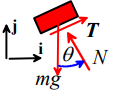3. Kinematics We can use the circular motion formula to write down the acceleration of tbe block (see section 3.1.3)

a = -rω2(cosθi+sinθj)

4. EOM: The equation of motion is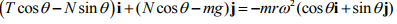5. Solution: The i and j components of the equation of motion can be solved for N and T – Mupad makes this painless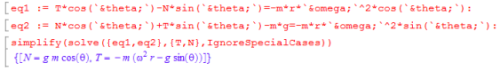To find the point where the block just starts to slip, we use the friction law. Recall that, at the point of slip

|T| = μN

For the block to slip with θ = 0

|-rω2| = μg

so the critical angular velocity is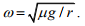Since the tangential traction T is negative, and the friction force must oppose sliding, the block must slide outwards, i.e. r is increasing during slip.

Offer running on EduRev: Apply code STAYHOME200 to get INR 200 off on our premium plan EduRev Infinity!

## Introduction to Dynamics and Vibrations- Notes, Videos, MCQs

20 videos|53 docs

,

,

,

,

,

,

,

,

,

,

,

,

,

,

,

,

,

,

,

,

,

;